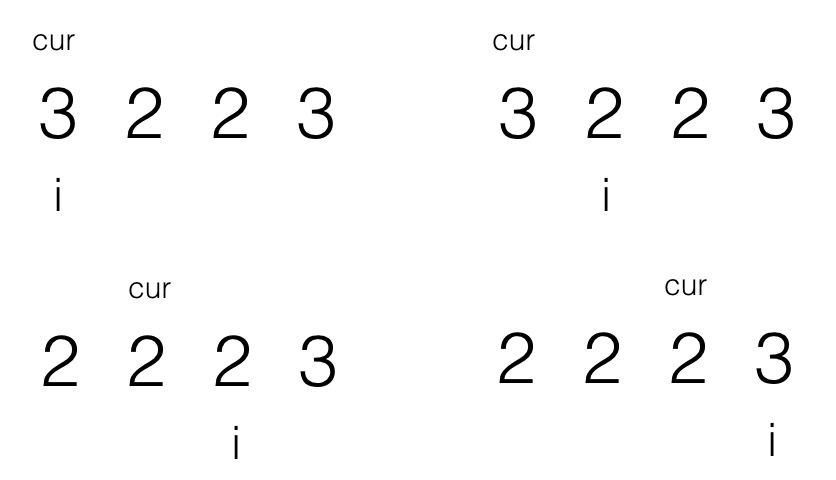Time Flying### 题目

``````给定 nums = [3,2,2,3], val = 3,

``````给定 nums = [0,1,2,2,3,0,4,2], val = 2,

### 解题

``````/**
* @param {number[]} nums
* @param {number} val
* @return {number}
*/
var removeElement = function (nums, val) {
let place
while (~nums.indexOf(val)) {
place = nums.indexOf(val)
nums.splice(place, 1)
}
return nums.length
}````````````/**
* @param {number[]} nums
* @param {number} val
* @return {number}
*/
var removeElement = function (nums, val) {
let cur = 0

for (let i = 0; i < nums.length; i++) {
if (nums[i] !== val) {
nums[cur] = nums[i]
cur++
}
}
return cur
}``````

26、80、283。